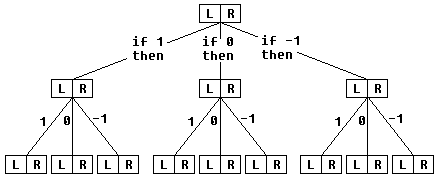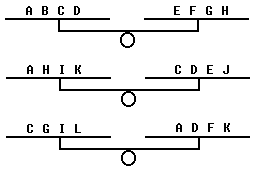# Thought Less Mathematics

This is an excerpt from
David Gale's Tracking the
Automatic Ant
, Springer, 1998## THOUGHT LESS MATHEMATICS

#### Donald J. Newman

One type of problem that we all "teethed on" in our mathematical youth was the so-called weighing problem. We learned therein the valuable lesson of "branching" procedures: if this and this happens, then we do that and that, but if it does not happen, then instead we do such and such.

These "branching" procedures emerged as a fundamental method in weighing problems, perhaps the right method for problems in general. Our minds were even tempted to go further. This might be the right path to follow for mathematics in general. And if it is right for mathematics, then it might be the right way to think altogether! WOW.

In an article in the New Yorker, Jeremy Bernstein pointed with admiration to the use of this branching reasoning as an index of real mathematical talent. The example he chose was the famous 12-coin problem, and the solver he pointed to was the then Harvard undergraduate Charles (Ariel) Zemach.

So, this branching reasoning appeared to be fundamental and nearly universal.

Our purpose, however, is to deflate this notion! We illustrate with the 12-coin problem itself and a few other examples and hope to convince the reader that this branching reasoning is perhaps never needed. Any time there is a solution using branching, there is another one that does not use it (and is, as a result, cleaner and simpler).

### The 12-Coin Problem

Twelve identical-looking coins are given, and we are told that one of them has a weight different from the other 11. The problem is to determine which coin it is and whether it is heavier or lighter, in only three weighings of these coins on a balance scale.

Note first that even to describe a solution after one has found it seems to require branching. The outcome of a weighing is that the left tray of the balance goes down or up or remains level. We encode these outcomes by 1, - 1, and 0 respectively. Each weighing involves picking a pair of subsets L and R of the same cardinality and putting them on the left and right trays, respectively. The "flow diagram" for a weighing procedure looks like this:where for each L and R one would have to list the elements of the appropriate subsets. Compare this to the following nonbranching instructions. Let the coins be labeled A, B, ..., L. Then doOne easily verifies that this works. Namely, if A is the phony coin and it is heavy, then the outcome will be 1, 1, - 1, and if it is light, the outcome will be - 1, - 1, 1. If B is the culprit and is heavy, then the outcome will be 1, 0, 0, and if it is light - but wait a minute. We do not have to go through all this. All we have to do is notice that no two coins have the same or opposite "itineraries"; that is, no two coins are always on the same tray or always on opposite trays. Therefore, for each of the 24 possible states (that is, which coin is counterfeit and whether it is heavy or light), there will be a different outcome. So given the Outcome, we will know the state. For example, if we know that the outcome is - 1, 0, 1, then the coin G must be heavy. Note, by the way, that it makes no difference in which order the weighings are performed. Also note that we can solve a slightly harder problem in which we allow the possibility that none of the coins is counterfeit, which win be true if and only if the outcome is 0, 0, 0.

But the question now is what sort of ingenuity was required to find these three weighings. The answer-none. We let the solution give itself. The real message we wish to impart then is that old, complicated, clever solution was a waste of effort, an incorrect attitude! The new solution reasons backward from the 27 outcomes to the 24 counterfeit possibilities. Here's how it goes.

First, we make a list of 12 different outcome vectors, such that no outcome and its negative are on the list. A simple way to do this is to list the lexicographically positive vectors in lexicographic order, as in the columns of the following table.

ABCDEFGHIJKL
000011111111
0111-1-1-100011
1-101-101-101-10

Now, for the procedure to work, we must have the same number of Is and - Is in each row. The bottom row is correct as it stands. Reversing the sign of column C fixes the middle row, and reversing columns F, H, J, and L takes care of the top row. So we have

ABCDEFGHIJKL
00001-11-11-111
01-11-11-10001-1
1-101-101-101-10

Now each row of the table corresponds to a weighing, namely, put the + Is on the left and - Is on the right-and there is it. Perform the weighings, write down the outcome, and read off the guilty coin from the table. (The capital letters of the tables differ by a permutation from the ones given earlier, but this clearly makes no difference.)

In some cases, nonbranching solutions are found easily. I have picked a number between I and 8 and you must guess it by asking three yes-or-no questions. Of course, everyone uses the branching, or "interactive," strategy of successive bisecting, but one need not do this. Why not just ask in advance these three questions, in any order. Is the number in the set {11, 2, 3, 4}? in the set {11, 2, 5, 6}? in the set {11, 3, 5, 7}? Of course, this would not work if the allowable question had to be of the form Is the number greater than x? The example shows what is going on generally. There is a set of possible states, and one wants to learn the true state. Every question, or weighing, or "experiment" gives a partition of this set. One defines the intersection of k partitions in the obvious way as the partition formed by all intersections of sets of the k partitions. Then the true state can be learned in n experiments without branching if and only if one can find n partitions whose intersection is the partition by singletons.### Weighing Coins, Balls, What Not ...Copyright © 1996-2018 Alexander Bogomolny

 64842833